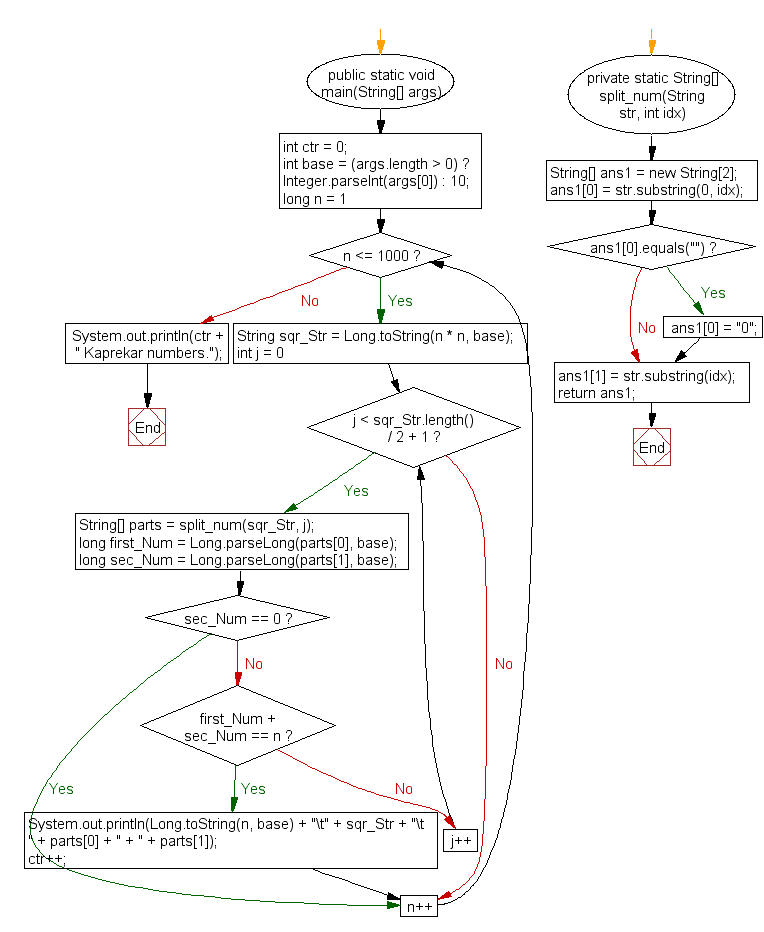﻿ Java exercises: Generate and show all Kaprekar numbers less than 1000 - w3resource# Java Exercises: Generate and show all Kaprekar numbers less than 1000

## Java Numbers: Exercise-4 with Solution

Write a Java program to generate and show all Kaprekar numbers less than 1000.

In number theory, a Kaprekar number for a given base is a non-negative integer, the representation of whose square in that base can be split into two parts that add up to the original number again. For instance, 45 is a Kaprekar number, because 452 = 2025 and 20 + 25 = 45.
The first few Kaprekar numbers in base 10 are:
1, 9, 45, 55, 99, 297, 703, 999, 2223, 2728, 4879, 4950, 5050, 5292, …

Pictorial Presentation:Sample Solution:

Java Code:

`````` public class Example4 {
public static void main(String[] args){
int ctr = 0;
int base = (args.length > 0) ? Integer.parseInt(args) : 10;
for(long n = 1; n <= 1000; n++){
String sqr_Str = Long.toString(n * n, base);
for(int j = 0; j < sqr_Str.length() / 2 + 1; j++){
String[] parts = split_num(sqr_Str, j);
long first_Num = Long.parseLong(parts, base);
long sec_Num = Long.parseLong(parts, base);
if(sec_Num == 0) break;
if(first_Num + sec_Num == n){
System.out.println(Long.toString(n, base) +
"\t" + sqr_Str + "\t  " + parts + " + " + parts);
ctr++;
break;
}
}
}
System.out.println(ctr + " Kaprekar numbers.");
}
private static String[] split_num(String str, int idx){
String[] ans1 = new String;
ans1 = str.substring(0, idx);
if(ans1.equals("")) ans1 = "0";
ans1 = str.substring(idx);
return ans1;
}
}
```
```

Sample Output:

```1       1         0 + 1
9       81        8 + 1
45      2025      20 + 25
55      3025      30 + 25
99      9801      98 + 01
297     88209     88 + 209
703     494209    494 + 209
999     998001    998 + 001
8 Kaprekar numbers.
```

Flowchart:Java Code Editor:

What is the difficulty level of this exercise?

﻿

## Java: Tips of the Day

Array vs ArrayLists:

The main difference between these two is that an Array is of fixed size so once you have created an Array you cannot change it but the ArrayList is not of fixed size. You can create instances of ArrayLists without specifying its size. So if you create such instances of an ArrayList without specifying its size Java will create an instance of an ArrayList of default size.

Once an ArrayList is full it re-sizes itself. In fact, an ArrayList is internally supported by an array. So when an ArrayList is resized it will slow down its performance a bit as the contents of the old Array must be copied to a new Array.

At the same time, it's compulsory to specify the size of an Array directly or indirectly while creating it. And also Arrays can store both primitives and objects while ArrayLists only can store objects.

Ref: https://bit.ly/3o8L2KH